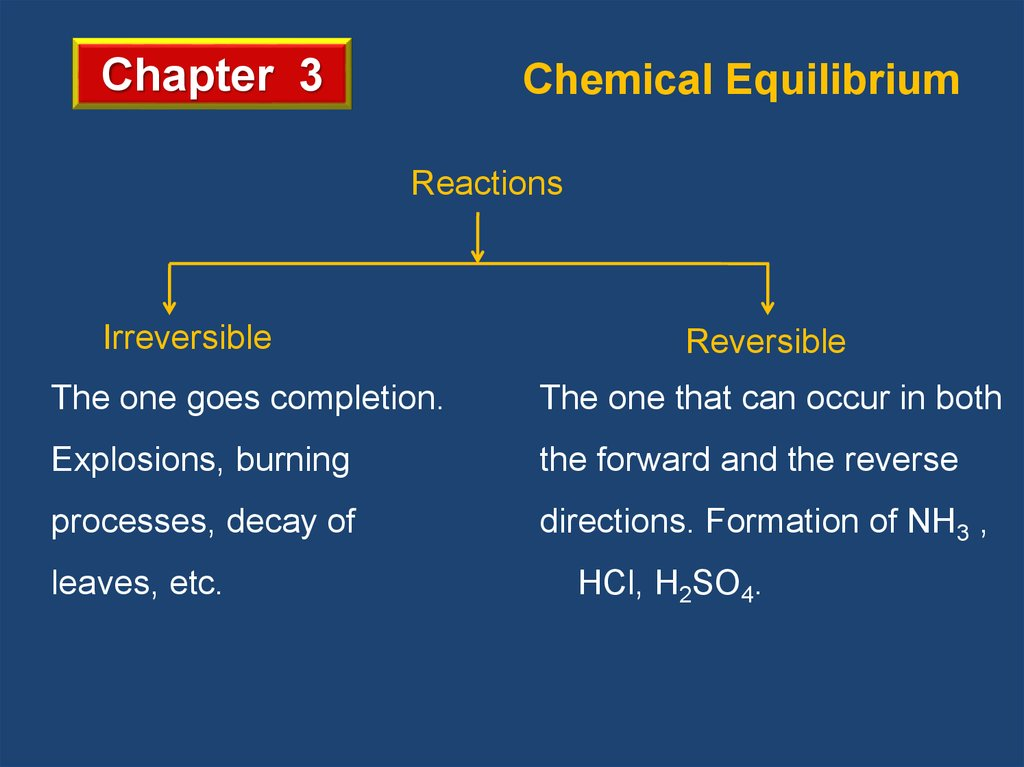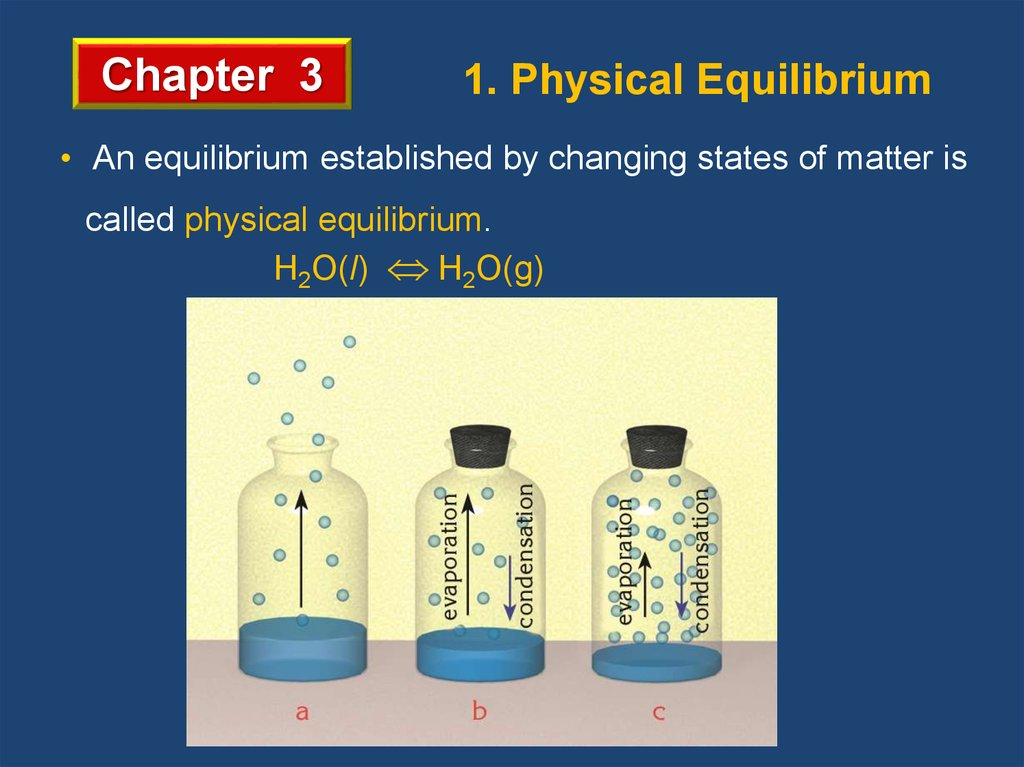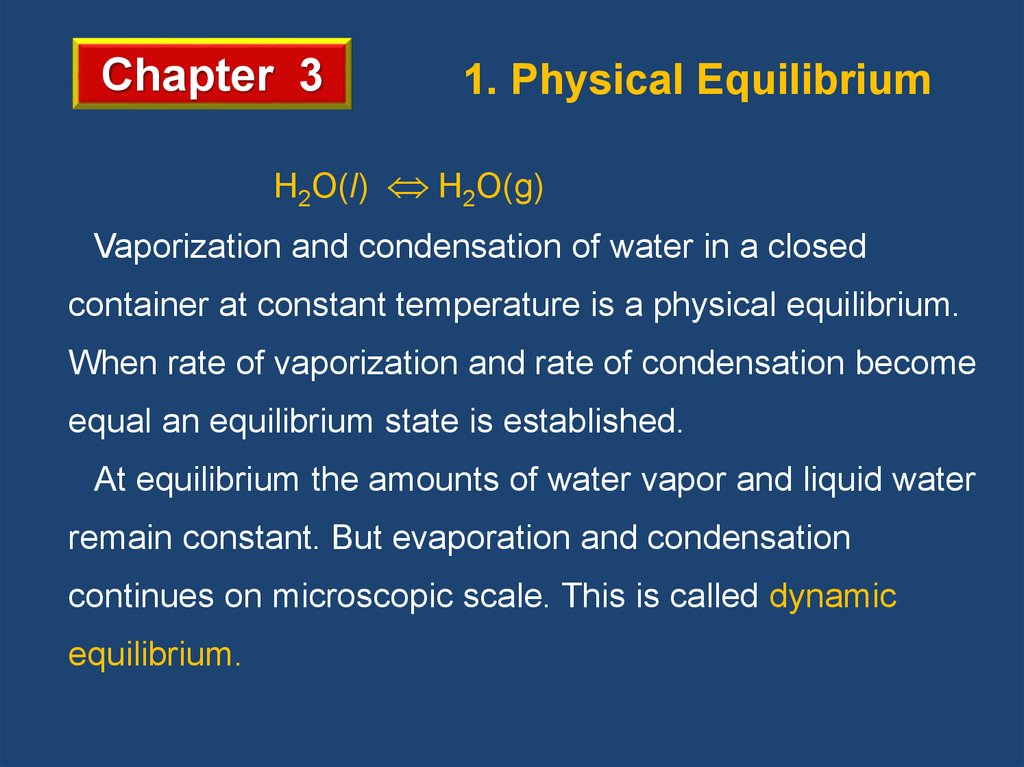# Chemical equilibrium. (Chapter 3)

## 1. Slayt 1

Chapter 3
Chemical Equilibrium
Reactions
Irreversible
Reversible
The one goes completion.
The one that can occur in both
Explosions, burning
the forward and the reverse
processes, decay of
directions. Formation of NH3 ,
leaves, etc.
HCl, H2SO4.

## 2. Slayt 2

Chapter 3
1. Physical Equilibrium
• An equilibrium established by changing states of matter is
called physical equilibrium.
H2O(l) H2O(g)

## 3. Slayt 3

Chapter 3
1. Physical Equilibrium
H2O(l) H2O(g)
Vaporization and condensation of water in a closed
container at constant temperature is a physical equilibrium.
When rate of vaporization and rate of condensation become
equal an equilibrium state is established.
At equilibrium the amounts of water vapor and liquid water
remain constant. But evaporation and condensation
continues on microscopic scale. This is called dynamic
equilibrium.

## 4. Slayt 4

Chapter 3
2. Chemical Equilibrium
• Chemical equilibrium is a state of balance in which the
rate of a forward reaction equals the rate of the reverse
reaction and the concentrations of products and reactants
remain unchanged.
2HI(g )
H2 (g ) I2 (g )
• Only a very small fraction of the collisions between H2
and I2 result in the formation of HI.
• As more HI molecules are made, they collide more often
and form H2 and I2 by the reverse reaction.

## 5. Slayt 5

Chapter 3
2. Chemical Equilibrium
• Rate Comparison for H2(g) + I2(g) 2HI(g)
• When the forward rate and the reverse rate are equal,
the system is at chemical equilibrium.

## 6. Slayt 6

Chapter 3
2. Chemical Equilibrium
• Rate Comparison for H2(g) + I2(g) 2HI(g)
Concentration, M
• When the reaction reaches
H2 or I2
equilibrium concentration of
substances become constant.
HI
teq
Time

## 7. Slayt 7

Chapter 3
2. Chemical Equilibrium
Fundamentals of Dynamic Equilibrium
• It does not show any visible change.
• It reaches through spontaneous change.
• Forward and reverse reactions take place continuously.
• It must be in a closed system.
• Temperature must be kept constant through equilibrium.
Example 1
a. H2(g) + Cl2(g) 2HCl(g)
(homogeneous)
b. Mg(s) + 2HCl(aq) MgCl2(aq) + H2(g)
(heterogeneous)

## 8. Slayt 8

Chapter 3 3. Factors Causing Equilibrium
There are two opposing factors causing equilibrium in a
chemical reaction;
1.Tendency toward minimum energy
In nature matter tend to lose energy to reach a lower
energy state.
• In exothermic reactions direction of minimum energy
is in favor of products.
• In endothermic reactions direction of minimum energy
is in favor of reactants.

## 9. Slayt 9

Chapter 3 3. Factors Causing Equilibrium
Example 2
Find the direction of minimum energy in the following
reactions.
a. N2H4(g) 2NO2(g) ∆H=+14 kcal
b. 2O3(g) 3O2(g) ∆H= - 68 kcal
c. 2NH3(g) + 22 kcal N2(g) + 3H2(g)
Solution
a. Reverse
b. Forward
c. Reverse

## 10. Slayt 10

Chapter 3 3. Factors Causing Equilibrium
2.Tendency toward maximum randomness (Entropy)
• Randomness increase from solid state to gaseous
state.
H2O(s) H2O(l) H2O(g)
Tendency toward maximum randomness
• Randomness is in favor of the side which has more
particles.
2O3(g) 3O2(g)
Tendency toward maximum randomness
• If two tendencies min. energy and max. randomness are in
opposite directions in a reaction the reaction can reach
equilibrium.

## 11. Slayt 11

Chapter 3 3. Factors Causing Equilibrium
Example 3
Find the following reactions whether they can reach
equilibrium or not.
a. N2H4(g) → 2NO2(g) ∆H=+14 kcal
b. Al(s) + 3HCl(aq) → AlCl3(aq) + 3/2H2(g) + 68 kcal
c. 2NH3(g) + 22 kcal → N2(g) + 3H2(g)
Solution
a. Yes
b. No
c. Yes

## 12. Slayt 12

4. Equilibrium Constant
Expression
Chapter 3
aA +
cC + dD
bB
Ratef = kf[A]a [B]b
Rater = kr[C]c [D]d
at equilibrium,
kf[A]a [B]b = kr[C]c [D]d
Ratef = Rater
kf
kr
c
[C] [D]
=
a
Kc =
b
[A] [B]
In general, Kc =
[C]c [D]d
d
[products]
[reactants]
[A]a [B]b

## 13. Slayt 13

Chapter 3
4. Equilibrium Constant
Expression
• Solid and liquid substances are not included in
Kc expressions.
Example 4
Write Kc expressions of the following
equilibrium reactions.
Solution
a. N2H4(g) 2NO2(g)
Kc =
b. 2Mg(s) + O2(g) 2MgO(s)
Kc =
c. 2NH3(g) N2(g) + 3H2(g)
Kc =
d. Ag+(aq) + Cl-(aq) AgCl(s)
Kc =
[NO2]2
[N2H4]
1
[O2]
[N2][H2]3
[NH3]2
1
[Ag+] [Cl-]

## 14. Slayt 14

4. Equilibrium Constant
Expression
Chapter 3
Example 7
For the equilibrium system described by:
PCl5 (g) PCl3 (g) + Cl2 (g)
Keq equals 35 at 487°C. If the concentrations of the PCl5 and
PCl3 at equilibrium are 0.015 M and 0.78 M, respectively, what
is the concentration of the Cl2?
Solution
Kc =
Kc =
[PCl3] [Cl2]
[PCl5]
(0.78) [Cl2]
(0.015)
= 35
[Cl2] = 0.67 M

## 15. Slayt 15

Chapter 3 5. Factors Affecting Equilibrium
(Le Chatelier’s Principle)
1. The Effect of Concentration
For the effect of concentration there are four cases:
Following reaction is given below;
N2(g) + 3H2(g) 2NH3(g)
at equilibrium at 25oC.
a. If some N2 or H2 is added to reaction container, the system
is disturbed and it will try to decrease this effect by moving
forward, then the reaction will reestablish a new equilibrium.
As a result of this, concentration of NH3 will increase.
b. If some NH3 is added the reaction will try to minimize this
effect by moving backward. As a result concentration of both
N2 and H2 will increase.

## 16. Slayt 16

Chapter 3 5. Factors Affecting Equilibrium
(Le Chatelier’s Principle)
1. The Effect of Concentration
N2(g) + 3H2(g) 2NH3(g)
at equilibrium at 25oC.
c. If some N2 or H2 is removed, decreasing concentration,
from reaction container, the system is disturbed and it will try
to decrease this effect by moving backward, then the reaction
will reestablish a new equilibrium. As a result of this,
concentration of NH3 will decrease.
d. If some NH3 is removed from the reaction container the
reaction will try to minimize this effect by moving forward. As
a result concentration of both N2 and H2 will decrease.

## 17. Slayt 17

Chapter 3
4. Equilibrium Constant
Expression
Example 17
The following reaction at equilibrium is given, at 25oC.
CO(g) + H2O(g)
CO2(g) +
H2(g).
Following changes are applied. Try to predict how the reaction
respond to the these effects.
c. Removal of H2O gas,
d. Removal of CO2 gas.

## 18. Slayt 18

Chapter 3
4. Equilibrium Constant
Expression
Solution
CO(g) + H2O(g)
CO2(g) +
H2(g).
a. The reaction shifts forward, and concentration of CO2 and H2
will increase and concentration of H2O will decrease.
b. The reaction shifts backward, and concentration of H2O and
CO will increase and concentration of CO2 will decrease.
c. The reaction shifts backward, and concentration of CO2 and
H2 will decrease and concentration of CO will increase.
d. The reaction shifts forward, and concentration of CO and
H2O will decrease and concentration of H2 will increase.

## 19. Slayt 19

Chapter 3 5. Factors Affecting Equilibrium
(Le Chatelier’s Principle)
2. Volume Changes or Effect of Pressure
• Change in volume alters the concentrations of gas reactants
and gas products.
• According to Le Chatelier’s principle, when the volume of the
system is decreased; the reaction shifts to the side having the
smaller number of coefficients of gases to decrease this effect.
• When the volume of the system is increased; the reaction shifts
to the side having the greater number of coefficients of gases to
decrease this effect.

## 20. Slayt 20

Chapter 3 5. Factors Affecting Equilibrium
(Le Chatelier’s Principle)
2. Volume Changes or Effect of Pressure
• For heterogeneous equilibrium the effect of volume is
predicted by counting the number of moles of gas indicated on
each side of an equation.
• Change in pressure affects the equilibrium reaction
conversely with respect to volume change.

## 21. Slayt 21

4. Equilibrium Constant
Expression
Chapter 3
Example 19
Consider the reaction:
2NH3(g)
3H2(g) +
N2(g)
Following changes are applied. Try to predict how the reaction
respond to the these effects.
a. Increasing volume,
b. Decreasing volume,
c. Increasing pressure.

## 22. Slayt 22

4. Equilibrium Constant
Expression
Chapter 3
Solution
2NH3(g)
3H2(g) +
2 mol gas reactant
N2(g)
4 mol gas product
a. The reaction shifts forward, and concentration of H2 and N2
will increase and that of NH3 will decrease.
b. The reaction shifts backward, and concentration of H2 and
N2 will decrease and that of NH3 will increase.
c. Increasing pressure means decreasing volume. Thus it will
have same effect in b.

## 23. Slayt 23

Chapter 3
4. Equilibrium Constant
Expression
Example 20
Consider the reaction:
CaCO3(s)
CO2(g) +
CaO(s)
Following changes are applied. How the reaction will the
reaction shift to reestablish equilibrium.
a. Increasing pressure,
b. Decreasing volume,
c. Increasing volume.

## 24. Slayt 24

Chapter 3
4. Equilibrium Constant
Expression
Solution
CaCO3(s)
0 mol gas reactant
CO2(g) +
CaO(s)
1 mol gas product
a. Increasing pressure means decreasing volume. The reaction
shifts backward.
b. The reaction shifts backward.
c. The reaction shifts forward.

## 25. Slayt 25

Chapter 3 5. Factors Affecting Equilibrium
3. Effect of Temperature
(Le Chatelier’s Principle)
• Effect of temperature can be
understood by knowing
whether the reaction is
exothermic or endothermic.
• In
endothermic reactions when temperature is increased the
reaction will proceed to forward to eliminate effect.
• In exothermic reactions when temperature is increased the
reaction will move backward to minimize effect.

## 26. Slayt 26

Chapter 3
4. Equilibrium Constant
Expression
Example 21
Consider the reaction:
2H2O(g)
2H2(g) +
O2(g)
ΔH = +136.6 kcal
Following changes are applied. How the reaction will the
reaction shift to reestablish equilibrium.
a. Increasing temperature,
b. Decreasing temperature.

## 27. Slayt 27

Chapter 3
4. Equilibrium Constant
Expression
Solution
2H2O(g)
2H2(g) +
O2(g)
ΔH = +136.6 kcal
The reaction is endothermic.
a. Forward reaction needs energy to take place, if
temperature is increased it will be in favor of forward
reaction. The reaction will shift forward.
b. Reverse reaction is exothermic. If temperature is
decreased it will be in favor of reverse reaction. The
reaction will shift backward.

## 28. Slayt 28

Chapter 3 5. Factors Affecting Equilibrium
(Le Chatelier’s Principle)
4. Effect of Catalyst
• Catalyst does not effect equilibrium reactions and Kc.
• They effect both forward and reverse reactions equally,
therefore they cause the reactions to reach equilibrium in a
shorter time.